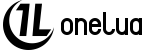math

## Functions

NUMBER math.minmax (NUMBER x, NUMBER min, NUMBER max)
Keeps a number between two limits. More...

NUMBER math.bitnot (NUMBER Bit)
Performs a bit logical negation (the 1 is converted to 0, and vice versa). More...

NUMBER math.bitand (NUMBER one, NUMBER two)
Performs a logic AND operation on each bit pair. More...

NUMBER math.bitor (NUMBER one, NUMBER two)
Performs a logic OR operation on each bit pair. More...

Math functions

## Function Documentation

 NUMBER math.minmax ( NUMBER x, NUMBER min, NUMBER max )

Keeps a number between two limits.

Parameters
 x The number to evaluate. min Lower limit. max Upper limit.
Returns
If x is greater than max, returns max. If x is less than min, returns min. If x is greater than min and less than max, returns x.
 NUMBER math.bitnot ( NUMBER Bit )

Performs a bit logical negation (the 1 is converted to 0, and vice versa).

Parameters
 Bit The number to process.
Returns
The number with operation applied.
 NUMBER math.bitand ( NUMBER one, NUMBER two )

Performs a logic AND operation on each bit pair.

Parameters
 one First number to process. two Second number to process.
Returns
The number with operation applied.
 NUMBER math.bitor ( NUMBER one, NUMBER two )

Performs a logic OR operation on each bit pair.

Parameters
 one First number to process. two Second number to process.
Returns
The number with operation applied.